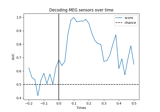# mne.decoding.PSDEstimator#

class mne.decoding.PSDEstimator(sfreq=6.283185307179586, fmin=0, fmax=inf, bandwidth=None, adaptive=False, low_bias=True, n_jobs=None, normalization='length', *, verbose=None)[source]#

Compute power spectral density (PSD) using a multi-taper method.

Parameters:
sfreq`float`

The sampling frequency.

fmin`float`

The lower frequency of interest.

fmax`float`

The upper frequency of interest.

bandwidth`float`

The bandwidth of the multi taper windowing function in Hz.

adaptive`bool`

Use adaptive weights to combine the tapered spectra into PSD (slow, use n_jobs >> 1 to speed up computation).

low_bias`bool`

Only use tapers with more than 90% spectral concentration within bandwidth.

n_jobs`int`

Number of parallel jobs to use (only used if adaptive=True).

normalization‘full’ | ‘length’

Normalization strategy. If “full”, the PSD will be normalized by the sampling rate as well as the length of the signal (as in Nitime). Default is `'length'`.

verbose

Control verbosity of the logging output. If `None`, use the default verbosity level. See the logging documentation and `mne.verbose()` for details. Should only be passed as a keyword argument.

Methods

 `fit`(epochs_data, y) Compute power spectral density (PSD) using a multi-taper method. `fit_transform`(X[, y]) Fit to data, then transform it. `transform`(epochs_data) Compute power spectral density (PSD) using a multi-taper method.
fit(epochs_data, y)[source]#

Compute power spectral density (PSD) using a multi-taper method.

Parameters:
epochs_data`array`, shape (n_epochs, n_channels, n_times)

The data.

y`array`, shape (n_epochs,)

The label for each epoch.

Returns:
selfinstance of `PSDEstimator`

The modified instance.

fit_transform(X, y=None, **fit_params)[source]#

Fit to data, then transform it.

Fits transformer to `X` and `y` with optional parameters `fit_params`, and returns a transformed version of `X`.

Parameters:
X`array`, shape (n_samples, n_features)

Training set.

y`array`, shape (n_samples,)

Target values or class labels.

**fit_params`dict`

Additional fitting parameters passed to the `fit` method..

Returns:
X_new`array`, shape (n_samples, n_features_new)

Transformed array.

transform(epochs_data)[source]#

Compute power spectral density (PSD) using a multi-taper method.

Parameters:
epochs_data`array`, shape (n_epochs, n_channels, n_times)

The data.

Returns:
psd`array`, shape (n_signals, n_freqs) or (n_freqs,)

The computed PSD.

## Examples using `mne.decoding.PSDEstimator`#Decoding (MVPA)

Decoding (MVPA)